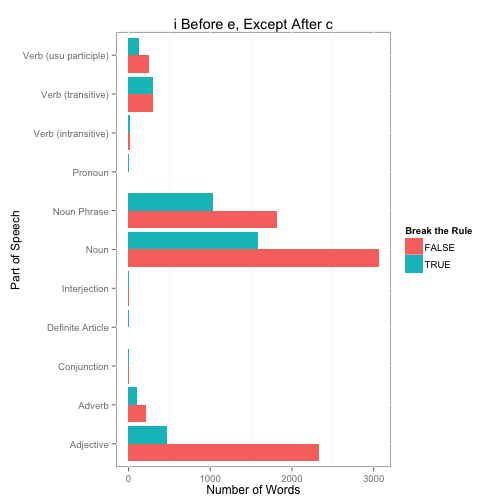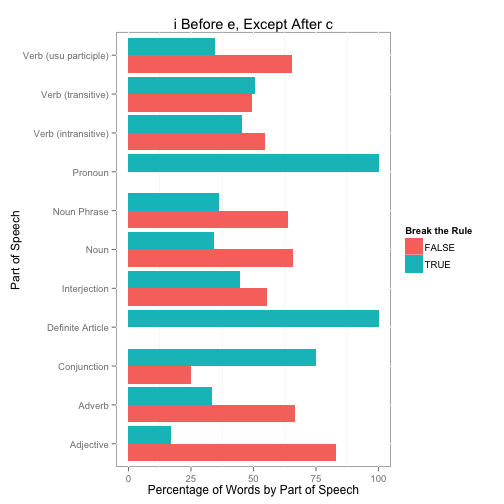# i Before e Except After c

When I went to school we were always taught the “i before e, except after c” rule for spelling. But how accurate is this rule? Kevin Marks tweeted today the following:

Not sure where he came up with that result, but seems simple enough to verify. First, download a English language word list compiled by Kevin Atkinson and available at SourceForge (I will use the Parts of Speech Database, or download my version from Github). I also create a data frame (from the README file) `partsOfSpeech` that maps the codes to descriptions that we will use later.

``````require(ggplot2)
require(reshape)

partsOfSpeech <- as.data.frame(matrix(c(
'N','Noun',
'P','Plural',
'h','Noun Phrase',
'V','Verb (usu participle)',
't','Verb (transitive)',
'i','Verb (intransitive)',
'A','Adjective',
'v','Adverb',
'C','Conjunction',
'P','Preposition',
'!','Interjection',
'r','Pronoun',
'D','Definite Article',
'I','Indefinite Article',
'o','Nominative'), ncol=2, byrow=TRUE), stringsAsFactors=FALSE)
names(partsOfSpeech) <- c('Code','Description')

words <- read.table('part-of-speech.txt', sep='\t', header=FALSE, quote='',
col.names=c('Word','POS'), stringsAsFactors=FALSE)
nrow(words)

##  295172
``````

The parts-of-speech is coded such that the letters before `|` character come from the original Moby database and letters after the `|` character come from WordNet. The first character corresponds to the primary classification. The following R code will split this field into two new variables, `Moby` and `WordNet`, and then strip the first character from `WordNet` to create a `WordNetPrimary` variable. We will use this classification later for plotting purposes.

``````tmp <- lapply(words\$POS, FUN=function(x) {
x <- unlist(strsplit(x, '|', fixed=TRUE) )
if(length(x) == 1) return(c(NA, x[]))
else if(x[] == '') return(c(NA, x[]))
else return(c(x[], x[]))
})
words\$Moby <- sapply(tmp, function(x) x)
words\$WordNet <- sapply(tmp, function(x) x)
words\$WordNetPrimary <- substr(words\$WordNet, 1, 1)
table(words\$WordNetPrimary, useNA='ifany')

##
##      !      A      C      D      h      i      N      p      P      r
##    260  51914     54     60  71566   2239 119441   8506     99     85
##      t      v      V   <NA>
##  12399  13730  12124   2695
``````

We use the `grep` function to get three vectors representing all the “ie”, “ei”, and “cei” words. We also print the number of each type word and the percentage of all words this represents.

``````ie <- grep('ie', words\$Word)
ei <- grep('ei', words\$Word)
cei <- grep('cei', words\$Word)
cie <- grep('cie', words\$Word)

length(ie); length(ie) / nrow(words) * 100

##  10647

##  3.607

length(ei); length(ei) / nrow(words) * 100

##  3542

##  1.2

length(cei); length(cei) / nrow(words) * 100

##  202

##  0.06843

length(cie); length(cie) / nrow(words) * 100

##  654

##  0.2216
``````

Number of words that follow the rule, “i before e except after c”

``````length(ie) + length(cei) - length(cie)

##  10195
``````

Number of i after e words that are not after c (first way to break the rule).

``````length(ei[!(ei %in% cei)])

##  3340
``````

Number of i before e words that are after c (the other way to break the rule).

``````length(cie)

##  654
``````

Percentage of words that break the rule.

``````(length(ei[!(ei %in% cei)]) + length(cie)) / sum(length(ie), length(ei)) * 100

##  28.15
``````

So of the 14,189 “ie” and “ei” words, 3,994 break the “i before e, except after c” rule, or about 28.1%.

Let’s see how this breaks out by part-of-speech.

``````thewords <- words[c(ie,ei),]
thewords\$BreakRule <- TRUE
thewords[which(row.names(thewords) %in% c(cei, ie[!(ie %in% cie)])),]\$BreakRule <- FALSE

#Counts
tab <- as.data.frame(table(thewords\$WordNetPrimary, thewords\$BreakRule, useNA='ifany'))
tab <- merge(tab, partsOfSpeech, by.x='Var1', by.y='Code', all.x=TRUE)

ggplot(tab, aes(x=Description, y=Freq, fill=Var2)) +
geom_bar(stat='identity', position='dodge') +
ylab('Number of Words') + xlab('Part of Speech') +
scale_fill_hue('Break the Rule') +
ggtitle('i Before e, Except After c') + coord_flip()
````````````#Percentages
tab2 <- as.data.frame(prop.table(table(thewords\$WordNetPrimary,
thewords\$BreakRule, useNA='ifany'), 1) * 100)
tab2 <- merge(tab2, partsOfSpeech, by.x='Var1', by.y='Code', all.x=TRUE)
ggplot(tab2, aes(x=Description, y=Freq, fill=Var2)) +
geom_bar(stat='identity', position='dodge') +
ylab('Percentage of Words by Part of Speech') + xlab('Part of Speech') +
scale_fill_hue('Break the Rule') +
ggtitle('i Before e, Except After c') + coord_flip()
``````A few last details. Here is the proportional table of words that break the rule by part-of-speech. Lastly, the definite article and pronoun words (three of each) that all break the rule.

``````cast(tab2, Description ~ Var2, mean, value='Freq')

##              Description FALSE   TRUE
## 1              Adjective 83.17  16.83
## 2                 Adverb 66.56  33.44
## 3            Conjunction 25.00  75.00
## 4       Definite Article  0.00 100.00
## 5           Interjection 55.56  44.44
## 6                   Noun 65.84  34.16
## 7            Noun Phrase 63.70  36.30
## 8                Pronoun  0.00 100.00
## 9    Verb (intransitive) 54.55  45.45
## 10     Verb (transitive) 49.42  50.58
## 11 Verb (usu participle) 65.45  34.55
## 12                  <NA> 67.26  32.74

thewords[which(thewords\$WordNetPrimary == 'D'), ]

##           Word POS Moby WordNet WordNetPrimary BreakRule
## 113927  either DCv <NA>     DCv              D      TRUE
## 182679 neither DCv <NA>     DCv              D      TRUE
## 262111   their   D <NA>       D              D      TRUE

thewords[which(thewords\$WordNetPrimary == 'r'), ]

##               Word POS Moby WordNet WordNetPrimary BreakRule
## 262112      theirs   r <NA>       r              r      TRUE
## 262113   theirself   r <NA>       r              r      TRUE
## 262114 theirselves  rp <NA>      rp              r      TRUE
``````

### Part II - Using only the 5,000 Most Frequently Used Words

Here is an update using the list of 5,000 most commonly used words from http://www.wordfrequency.info/top5000.asp (note there really are only 4,354 unique words since the same word can be used in different parts-of-speech). Of the 4,354 unique words, 96, or about 2.2%, have an “ie” or “ei” in the word. Of those 96 words, 31, or 32.3% break the “i before e except after c” rule.

``````words <- read.csv('MostUsedWords.csv')
dups <- words[words\$Word %in% words[duplicated(words\$Word),]\$Word,]
head(dups[order(dups\$Word),])

##      Rank  Word Part.of.speech Frequency Dispersion
## 47     46 about              i    874406       0.96
## 180   179 about              r    208550       0.97
## 897   896 above              i     44130       0.95
## 1604 1599 above              r     23866       0.92
## 1553 1548 abuse              n     24534       0.93
## 3783 3778 abuse              v      7554       0.94

length(unique(words\$Word))

##  4354

words <- words[!duplicated(words\$Word),]

ie <- grep('ie', words\$Word)
ei <- grep('ei', words\$Word)
cei <- grep('cei', words\$Word)
cie <- grep('cie', words\$Word)

#Percentage of words that break the rule.
(length(ei[!(ei %in% cei)]) + length(cie)) / sum(length(ie), length(ei)) * 100

##  32.29
``````

### Part III - Weighted by Frequency of Words

Using the same list as part II above, let’s consider the word frequency. That is, we’ll weight each word by it’s frequency according to WordFrequency.info. Using this approach, 47% of “ie” words break the rule. Put another way, for each “ie” word you encounter reading, there is a 47% chance it does not follow the “i before e, except after c” rule.

``````words <- read.csv('MostUsedWords.csv')
ie <- grep('ie', words\$Word)
ei <- grep('ei', words\$Word)
cei <- grep('cei', words\$Word)
cie <- grep('cie', words\$Word)
(sum(words[ei[!(ei %in% cei)],'Frequency']) + sum(words[cie,'Frequency'])) /
sum(words[ie,'Frequency'], words[ei,'Frequency']) * 100

##  46.81
``````

### Related

comments powered by Disqus